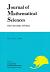## Vol. 15 (2008) No. 04Journal of Mathematical Sciences The University of Tokyo

1. BENMERIEM, Khaled; BOUZAR, Chikh
Ultraregular generalized functions of Colombeau type
Vol. 15 (2008), No. 4, Page 427--447.

2. Prokhorov, Yuri
Gap conjecture for $3$-dimensional canonical thresholds
Vol. 15 (2008), No. 4, Page 449--459.

3. Sato, Yoshihisa
2-spheres of square $-1$ and the geography of genus-2 Lefschetz fibrations
Vol. 15 (2008), No. 4, Page 461--491.
Quantization of differential systems with the affine Weyl group symmetries of type $C_N^{(1)}$
Exact power series in the asymptotic expansion of the matrix coefficients with the corner $K$-type of $P_J$-principal series representations of $Sp(2,\R)$